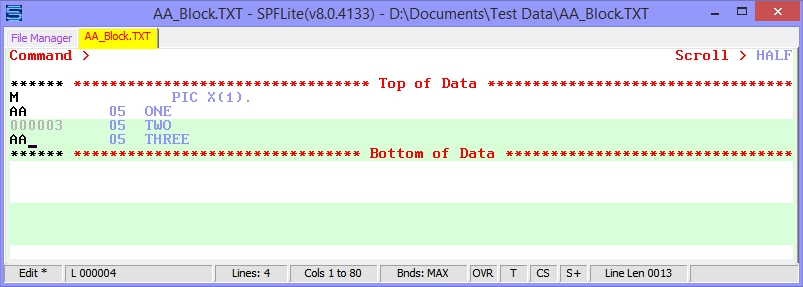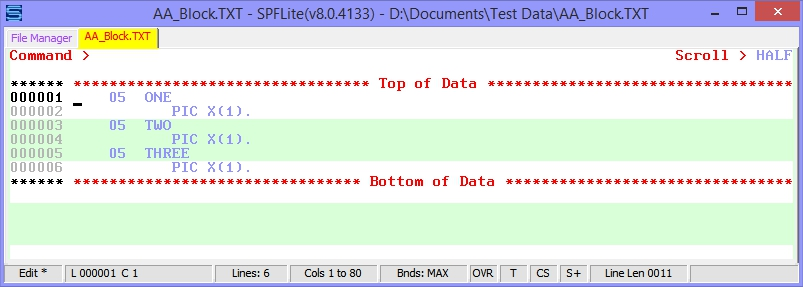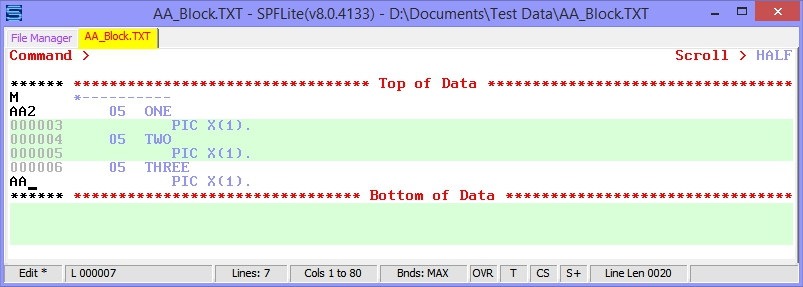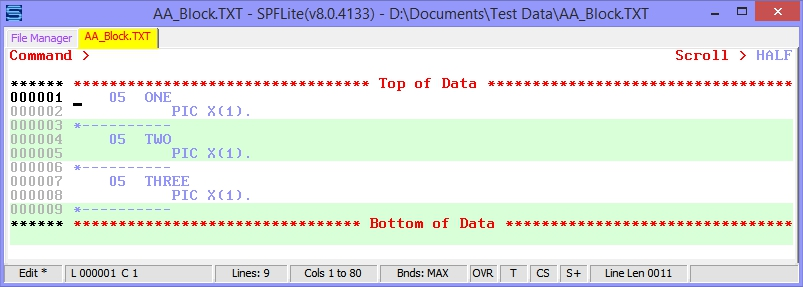Syntax

 AA[n] / AA

Operands

 n A number that tells the editor to repeat the associated line command or COPY or PASTE command after every nth line in the AA block. If you do not type a number, or if the number you type is 1, the editor performs the command after each line in the range.

Description

The AA block command is used to copy or insert after every line in a block of lines. If a line or group of lines is to be inserted after (or before) every line in a range of lines, standard ISPF requires the inserted data to be manually placed by individual copy and A (or B) line commands. SPFLite will allow definition of an AA block, and then insertion of data will occur after every line in that block.

If a number n follows the AA, it means to copy after every nth line. If n is omitted it defaults to a value of 1, meaning 'after every line'.

The value of n is used to determine the insertion points where moved or copied lines are to be placed. Insertion points are determined by going forwards and treating the initial AA block command as the starting point.

The examples below are shown using a M line command. AA can be used with C/CC or M/MM or the Primary commands COPY and PASTE as well.

As an example, consider an AA block. Assume that the first AA command is on “relative line 1”. If n is omitted, then lines are copied or moved after every line in the block:

Before:After:If n is present, then lines are copied or moved after “relative line n”, “relative line 2n”, “relative line 3n”, etc. Here, note that the first AA command is on line 2, which is “relative line 1”, and “relative line n” where n=2 is line 3. So, the insertion points are after lines 3, 5 and 7:

Before:After:Created with the Personal Edition of HelpNDoc: Free Qt Help documentation generator